# Finding Zeros, Roots, Solutions, x-intercepts

Zeros or roots of functions and solutions to equations can either be real or complex numbers. x-intercepts can only be real numbers.

The TI graphing calculator can be used to find the real roots of an equation.

## All calculators

1. If your equation has something on the right side, subtract it from both sides so that the right hand side is 0.
2. Go to Y=
3. Enter the expression
4. Hit Graph
5. Change the viewing window if necessary so that you can see where the graph crosses the x-axis. You need to make sure that there is enough to the left and right of the x-intercept to select a point.
6. Continue on to the specific steps for your calculator. If there is more than one x-intercept, you may need to change your viewing window and repeat the steps for each x-intercept.

## TI-82

1. Press Calc (2nd Trace)
2. Choose Root (#2)
3. For the Lower Bound, arrow to the left of the x-intercept and press enter.
4. For the Upper Bound, arrow to the right of the x-intercept and press enter.
5. For the Guess, arrow to the x-intercept and press enter.
6. The TI-82 will return a value for x and 0 (or really close to it) for y.

## TI-83

For the TI-83, you may enter left bounds, right bounds, and guesses from the keyboard instead of arrowing and hitting enter. When it asks for a value, just enter your number and press enter.

1. Press Calc (2nd Trace)
2. Choose Zero (#2)
3. For the Left Bound, arrow to the left of the x-intercept and press enter.
4. For the Right Bound, arrow to the right of the x-intercept and press enter.
5. For the Guess, arrow to the x-intercept and press enter.
6. The TI-83 will return a value for x and 0 (or really close to it) for y.

## TI-85 / TI-86

1. Press Graph
2. Press More and then Math (F1)
3. Press Lower (F1). Arrow to the left of the x-intercept and press enter.
4. Press Upper (F2). Arrow to the right of the x-intercept and press enter.
5. Press Root (F3). Arrow to the x-intercept and press enter.
6. The TI-85 will return a value for x and 0 (or really close to it) for y.

## TI-89 / TI-92

For the TI-89 and TI-92, you may enter lower bounds or upper bounds from the keyboard instead of arrowing and hitting enter. When it asks for a value, just enter your number and press enter.

1. Press Math (F5)
2. Choose Zero (#2)
3. For the Lower Bound, arrow to the left of the x-intercept and press enter.
4. For the Upper Bound, arrow to the right of the x-intercept and press enter.
5. The TI-89 will return a value for x and 0 for y.

You do not need to supply a guess for the TI-89. You should make sure there is only one x-intercept in the interval specified or you won't know which one it is going to give you.

## Example (TI-83+)

Find all real solutions to the equation exp(-x2) = -x.

Note: exp(x) is the exponential function and is often written as ex. It is ex (2nd LN) on the calculator, but gets displayed as e^(x).

Begin by moving the -x to the left side where it becomes +x. The function to graph is y1 = x + e^(-x2).

Graph Zero Left Right Guess Solution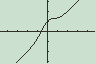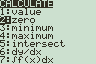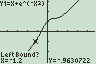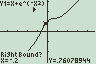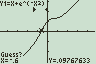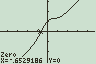The calculator says the solution is x = -0.6529186. The y-value of 0 is ignored. There was no y in the original problem.